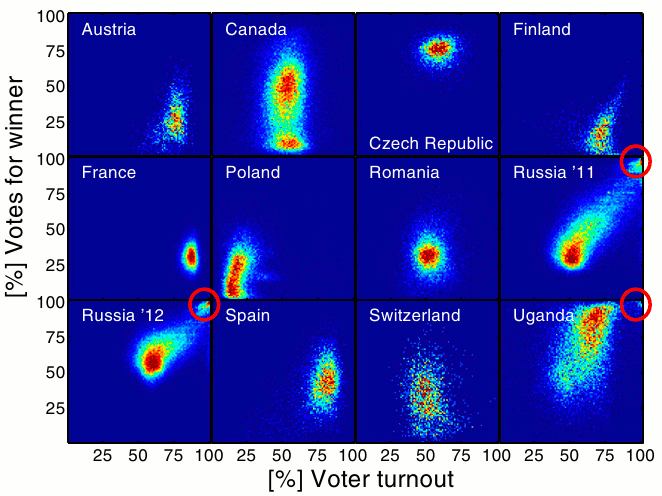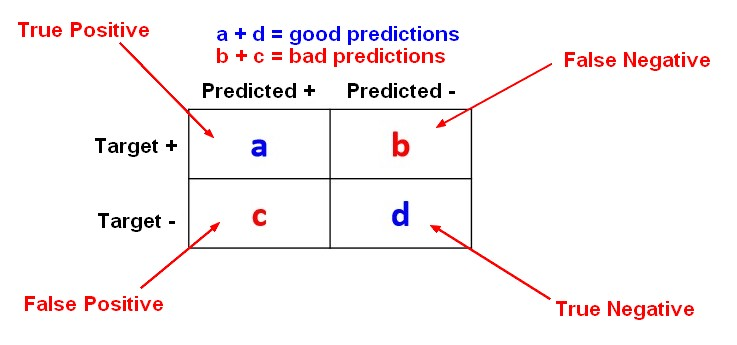# Statistics - ROC Plot and Area under the curve (AUC)

The Area Under Curve (AUC) metric measures the performance of a binary classification.

In a regression classification for a two-class problem using a probability algorithm, you will capture the probability threshold changes in an ROC curve.

Normally the threshold for two class is 0.5. Above this threshold, the algorithm classifies in one class and below in the other class.

You may want to move this threshold. False positives (legitimate emails erroneously predicted as spam) are likely to cause more harm than false negatives (spam emails that are not identified as spam), as we might miss an important email, while it is easy to delete a spam message. In this case, we could require a higher threshold (probability) that a message is spam before we move it into a spam folder.

ROC means Receiver Operating Characteristic. It's an historical term from WW2 that was used to measure the accuracy of radar operators.

This is a single curve that captures the behaviour of the classification rate when varying the classification threshold.

## Problem description

The goal is to have:

## Curve

With a true positive rate of one and a false positive rate of zero, the best curve will right up as far as possible into the top left hand corner.

The 45 degree line is the kind of no information line (ie random See ROC curve with sensitivity).

### Sensitivity / Specificity

See Sensitivity and Specificity

## Tools

### Weka

A ROC curve for a J48 algorithm.

Discover MoreData Mining - (Anomaly|outlier) Detection

The goal of anomaly detection is to identify unusual or suspicious cases based on deviation from the norm within data that is seemingly homogeneous. Anomaly detection is an important tool: in data...Data Mining - Lift Chart

The ROC chart is similar to the gain or lift charts in that they provide a means of comparison between classification models. Model Evaluation - Classification...Machine Learning - Area under the curve (AUC)

The Area under the curve (AUC) is a performance metrics for a binary classifiers. By comparing the ROC curves with the area under the curve, or AUC, it captures the extent to which the curve is up in the...Statistics Learning - (Error|misclassification) Rate - false (positives|negatives)

The error rate is a prediction error metrics for a binary classification problem. The error rate metrics for a two-class classification problem are calculated with the help of a Confusion Matrix. The...